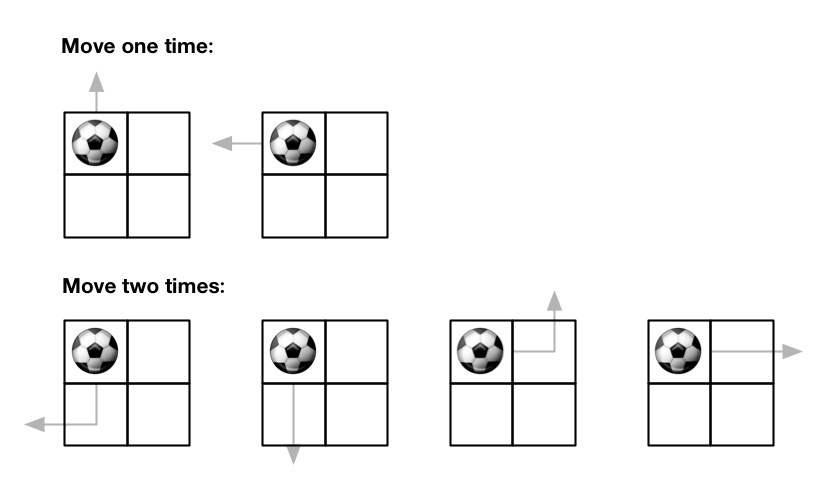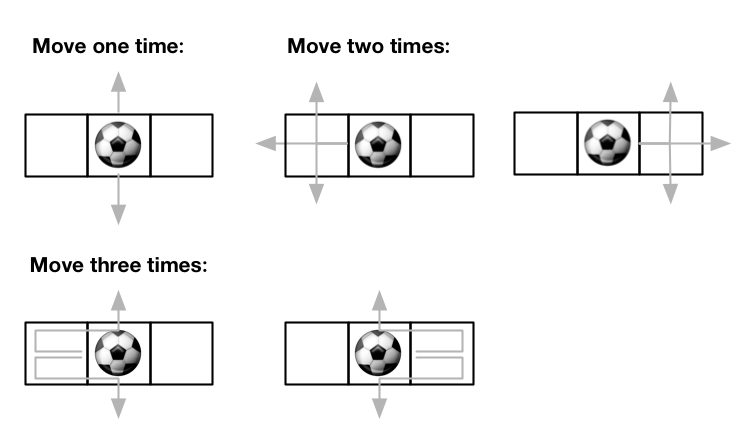576. Out of Boundary Paths
Medium
3K
232

There is an `m x n` grid with a ball. The ball is initially at the position `[startRow, startColumn]`. You are allowed to move the ball to one of the four adjacent cells in the grid (possibly out of the grid crossing the grid boundary). You can apply at most `maxMove` moves to the ball.

Given the five integers `m`, `n`, `maxMove`, `startRow`, `startColumn`, return the number of paths to move the ball out of the grid boundary. Since the answer can be very large, return it modulo `109 + 7`.

Example 1:```Input: m = 2, n = 2, maxMove = 2, startRow = 0, startColumn = 0
Output: 6
```

Example 2:```Input: m = 1, n = 3, maxMove = 3, startRow = 0, startColumn = 1
Output: 12
```

Constraints:

• `1 <= m, n <= 50`
• `0 <= maxMove <= 50`
• `0 <= startRow < m`
• `0 <= startColumn < n`
Accepted
116.6K
Submissions
263.5K
Acceptance Rate
44.3%

Seen this question in a real interview before?
1/4
Yes
No

Discussion (0)

Related Topics